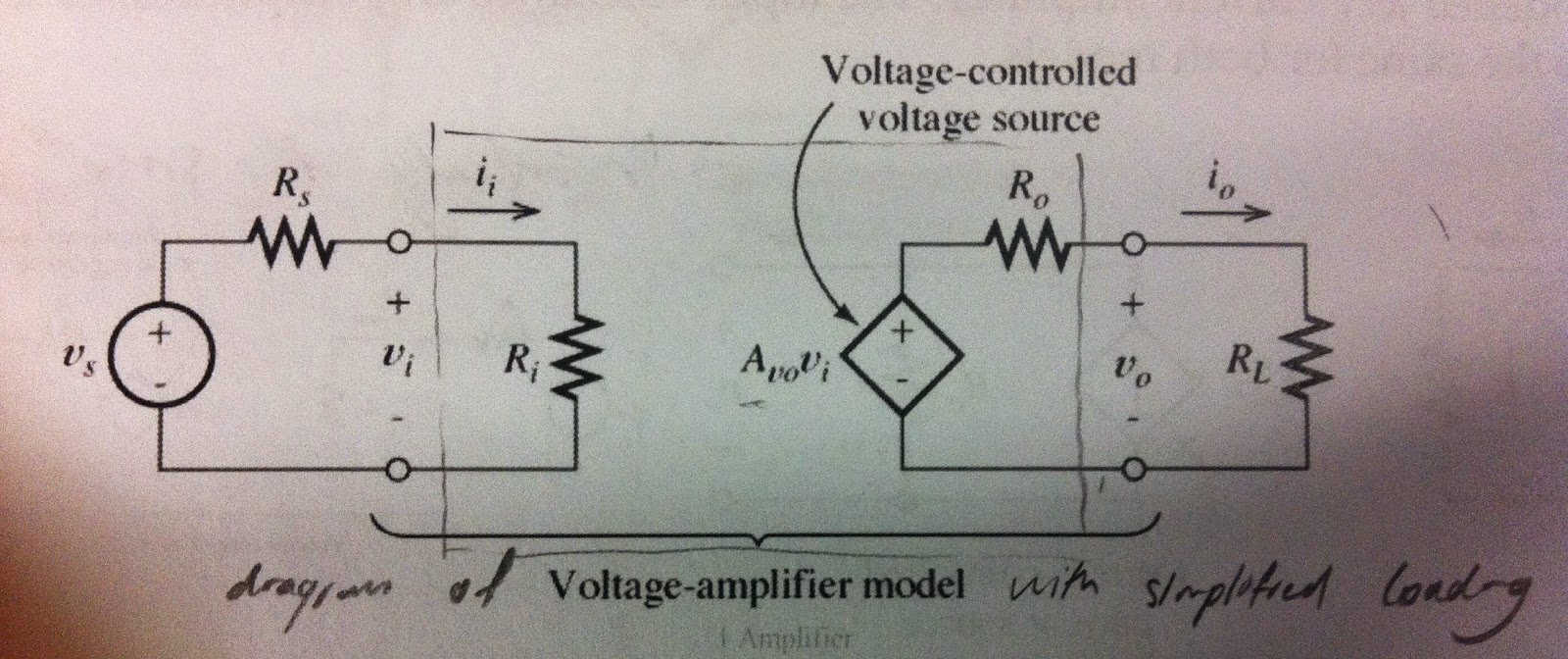## Introduction to Operational Amplifiers (Op-Amps)

In summary, the op-amp is a voltage amplifier, typically with two inputs, and a single output. For the sake of simplification, most op-amps can be simplified into the following model.http://en.wikipedia.org/wiki/Operational_amplifier
There are 5 pins;
V+ This is the noninverting input
V- This is the inverting input
Vout This is the output terminal
Vs+ This pin is attached to a high voltage source
Vs- This is the grounding pin

However, usually they are drawn as followshttp://hyperphysics.phy-astr.gsu.edu/

We do not explicitly show the power-supply connections (Vs+ and Vs-) in circuit diagrams.
It is assumed that the Vout voltage is made in relation to the circuit's ground.

The op-amp multiplies the difference between V+ and V-, and multiplies it by Aol, the open loop gain.
i.e. Vout = |(V+ - V-)| x Aol

This gain parameter, Aol is very large, and is assumed to be infinite, in the case of an ideal op-amp.
For the simplicity of calculation, we simply assume that, because Vout is real,
Vout/Aol = |V+ - V-|

i.e. V+ = V-

This concept is known as the summing point constraint.
This is the default assumption we make whenever calculating feedback loops for ideal op-amps.
By making this assumption, we can apply standard circuit analysis principles.
While there is a difference between V+ and V-, we have to assume that they are the same value for the sake of calculation, such as the following cases demonstrated below.

## Using the Op-Amp in circuits

Inverting amplifiers - negative feedback
Inverting amplifiers - positive feedback
Noninverting amplifiers
Non inverting amplifiers always have negative feedback.

## Amplifier models:

There exist four amplifier models;
1. Voltage Amplifier Model
2. Current Amplifier Model
3. Transconductance Amplifier Model
4. Transresistance Amplifier ModelSimplified amplifier model

To begin, we need to understand the voltage amplifier model.

### Voltage amplifier model

Consider the image above. In the case of the voltage amplifier model, the internal components can be modelled as the following;

Where
Vi = input voltage
Vo = output voltage
Ii = input current
Io = output current
Ri = input resistance/impedance
Ro = output resistance/impedance

The gain property of this model is represented by a voltage-controlled voltage source.

Note:
By definition, voltage gain, Av = Vo/Vi

Open-circuit voltage gain = Avo
(if the load is an open circuit, as shown above, there is no drop across the output resistance, and Vo = Avo*Vi)

Given a model with external loading (i.e. with external components attached), the model can be simplified as follows, for analysis.Aside from voltage gain. there also exists current gain, Ai, which can be calculated as follows;
Ai = Io/Ii = (Vo/Rl)/(Vi/Ri) = Av*(Ri/Rl)

### Current amplifier model

Another method of modelling the amplifier is the current amplifier model.
This can be modelled as the following;

The gain property of this model is represented by a current-controlled current source.

Note:
By definition, current gain Ai = Io/Ii

Open-circuit current gain = Aisc
(If the load is a short circuit, no current flows through resistor Ro, and Io/Ii is short-circuit current gain.)

### Transconductance amplifier model

Another method of modelling the amplifier is the transconductance-amplifier model.
This can be modelled as the following;

The gain property of this model is represented by a voltage-controlled current source.

Observe that while the transconductance-amplifier and current-amplifier models use the Norton equivalent circuit, the voltage-amplifier model uses the Theverin equivalent circuit, as will the transresistance model, as will be demonstrated below.

### Transresistance amplifier model

Another method of modelling the amplifier is the transresistance-amplifier model.
This can be modelled as the following;

The gain property of this model is represented by a current-controlled voltage source.

### Ideal amplifiers

If the reasons for the above table remain unknown, consider the following

Inputs:
The voltage and transconductance models are voltage controlled.
Thus, the input impedance Ri is ideally large, this maximises the voltage drop Vi to be maximised over Ri. (See earlier diagrams)

The current and transresistance models are current controlled.
Thus, to maximise the input current Ii, Ri is minimised.

Outputs:
The voltage and transresistance models are voltage sources.
Thus, to maximise the output Vo, the output impedance Ro is minimised. By doing so, the voltage drop over Rl is maximised.

The current and transconductance models are current sources.
Thus, to maximise the output current Io, the output impedance Ro is maximised, allowing the current to flow out of the amplifier (Ro becomes akin to an open circuit).

(Note that this logic holds because for the voltage sources, a Theverin equivalent circuit is used, while for the current sources, a Norton equivalent circuit is used. More information on Theverin and Norton can be found here.)

Hopefully, this provides an introduction to amplifier models. If you feel that any part of this post needs improvements, feel free to contact us at engyfun@gmail.com

References:
Kroll, (2009). 1st ed. [ebook] Available at: http://surf.hep.upenn.edu/~kroll/p364_fall09/handouts/simple_voltage_amplifier.pdf [Accessed 5 Mar. 2015].